# The 15 Most Famous Transcendental Numbers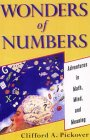I am in love with the mysterious transcendental numbers. Did you know that there are "more" transcendental numbers than the more familiar algebraic ones? Even so, only a few classes of transcendental numbers are known to humans, and it's very difficult to prove that a particular number is transcendental. In 1844, math genius Joseph Liouville (1809-1882) was the first to prove the existence of transcendental numbers. (More precisely, he was the first to prove that a specific number was transcendental.) Hermite proved that the number e was transcendental in 1873. Lindeman proved that pi was transcendental in 1882. For more information, see my book Wonders of Numbers from which this is excerpted.

Pickover Navigation Bar: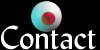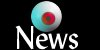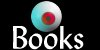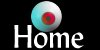The mathematical constant pi represents the ratio of the circumference of a circle to its diameter. It is the most famous ratio in mathematics both on Earth and probably for any advanced civilization in the universe. The number pi, like other fundamental constants of mathematics such as e = 2.718..., is a transcendental number. The digits of pi and e never end, nor has anyone detected an orderly pattern in their arrangement. Humans know the value of pi to over a trillion digits.

Transcendental numbers cannot be expressed as the root of any algebraic equation with rational coefficients. This means that pi could not exactly satisfy equations of the type: pi2 = 10, or 9pi4 - 240pi2 + 1492 = 0. These are equations involving simple integers with powers of pi. The numbers pi and e can be expressed as an endless continued fraction or as the limit of an infinite series. The remarkable fraction 355/113 expresses pi accurately to six decimal places.

In 1882, German mathematician F. Lindemann proved that pi is transcendental, finally putting an end to 2,500 years of speculation. In effect, he proved that pi transcends the power of algebra to display it in its totality. It can't be expressed in any finite series of arithmetical or algebraic operations. Using a fixed-size font, it can't be written on a piece of paper as big as the universe.

I also talk about all the mysteries of pi in my book Keys to Infinity.

Many of you have probably heard of pi and e. But are there other famous transcendental numbers? After conducting a brief survey of readers, I made a list of the fifteen most famous transcendental numbers. Can you list these in order of relative fame and/or usage?

1. pi = 3.1415 ...
2. e = 2.718 ...
3. Euler's constant, gamma = 0.577215 ... = lim n -> infinity > (1 + 1/2 + 1/3 + 1/4 + ... + 1/n - ln(n)) (Not proven to be transcendental, but generally believed to be by mathematicians.)
4. Catalan's constant, G = sum (-1)^k / (2k + 1 )^2 = 1 - 1/9 + 1/25 - 1/49 + ... (Not proven to be transcendental, but generally believed to be by mathematicians.)
5. Liouville's number 0.110001000000000000000001000 ... which has a one in the 1st, 2nd, 6th, 24th, etc. places and zeros elsewhere.
6. Chaitin's "constant", the probability that a random algorithm halts. (Noam Elkies of Harvard notes that not only is this number transcendental but it is also incomputable.)
7. Chapernowne's number, 0.12345678910111213141516171819202122232425... This is constructed by concatenating the digits of the positive integers. (Can you see the pattern?)
8. Special values of the zeta function, such as zeta (3). (Transcendental functions can usually be expected to give transcendental results at rational points.)
9. ln(2).
10. Hilbert's number, 2(sqrt 2 ). (This is called Hilbert's number because the proof of whether or not it is transcendental was one of Hilbert's famous problems. In fact, according to the Gelfond-Schneider theorem, any number of the form ab is transcendental where a and b are algebraic (a ne 0, a ne 1 ) and b is not a rational number. Many trigonometric or hyperbolic functions of non-zero algebraic numbers are transcendental.)
11. epi
12. pie (Not proven to be transcendental, but generally believed to be by mathematicians.)
13. Morse-Thue's number, 0.01101001 ...
14. ii = 0.207879576... (Here i is the imaginary number sqrt(-1). Isn't this a real beauty? How many people have actually considered rasing i to the i power? If a is algebraic and b is algebraic but irrational then ab is transcendental. Since i is algebraic but irrational, the theorem applies. Note also: ii is equal to e(- pi / 2 ) and several other values. Consider ii = e(i log i ) = e( i times i pi / 2 ) . Since log is multivalued, there are other possible values for ii.
Here is how you can compute the value of ii = 0.207879576...
1. Since e^(ix) = Cos x + i Sin x, then let x = Pi/2.
2. Then e^(iPi/2) = i = Cos Pi/2 + i Sin Pi/2; since Cos Pi/2 = Cos 90 deg. = 0. But Sin 90 = 1 and i Sin 90 deg. = (i)*(1) = i.
3. Therefore e^(iPi/2) = i.
4. Take the ith power of both sides, the right side being i^i and the left side = [e^(iPi/2)]^i = e^(-Pi/2).
5. Therefore i^i = e^(-Pi/2) = .207879576...
15. Feigenbaum numbers, e.g. 4.669 ... . (These are related to properties of dynamical systems with period-doubling. The ratio of successive differences between period-doubling bifurcation parameters approaches the number 4.669 ... , and it has been discovered in many physical systems before they enter the chaotic regime. It has not been proven to be transcendental, but is generally believed to be.)
Long ago, Keith Briggs from the Mathematics Department of the University of Melbourne in Australia computed what he believed to be the world-record for the number of digits for the Feigenbaum number:
4. 669201609102990671853203820466201617258185577475768632745651 343004134330211314737138689744023948013817165984855189815134 408627142027932522312442988890890859944935463236713411532481 714219947455644365823793202009561058330575458617652222070385 410646749494284981453391726200568755665952339875603825637225
Briggs carried out the computation using special-purpose software designed by David Bailey of NASA Ames running on an IBM RISC System/6000. The computation required a few hours of computation time.

Today, we know far more digits for the Feigenbaum constant. See this page for more than 10,000 digits. Related fascinating information can be found here.

### Ants and Transcendental NumbersImagine a race of talking ants. The ants can compress the infinite digits of pi in an interesting way. For example, let us imagine that the ants can speak by manipulating their crude jaws. The first ant in the long parade of ants screams out the first digit, "3". The next yells the number on its back, a "1". The next yells a "4", and so on. Further imagine that each ant speaks its digit in only half the time of the preceding ant. Each ant has a turn to speak. Only the most recent digit is spoken at any instant. If the first digit of pi requires 30 seconds to speak (due to the ant's cumbersome jaws and little brain), might the entire ant colony will speak all the digits of pi in a minute? (Again, this is because the infinite sum 1/2 minute + 1/4 minute + 1/8 minute + ... is equal to 1 minute.) Astoundingly, at the end of the minute, there will be a quick-talking ant that will actually say the "last" digit of pi! The geometer God, upon hearing this last digit, may cry, "That's impossible, because pi has no last digit!"
(See below for an email from Brian B. on the talking ants.)

Here are some nice web pages on transcendental numbers: 1, 2, and 3.

Here is a book on transcendental numbers.

### Dottie Number

Dottie number is the unique real root of cosx = x (namely, the unique real fixed point of the cosine function), which is 0.739085...Dottie noticed that whenever she typed a number into her calculator and pressed the cosine button repeatedly, the result always converged to this value. Wow. The number is well-known, having appeared in numerous elementary works on algebra already by the late 1880s. The Dottie number is transcendental as a consequence of the the Lindemann-Weierstrass theorem. This text about Dottie is adpoted from MathWorld.

### Comments from Colleagues on Their Passion for Transcendental Numbers

Although they are not often recognized as such even by mathematicians, there are a lot of commonly-used numbers that are also transcendental, which can easily be shown by the Gelfond-Schneider theorem mentioned in the tenth item on the list. If a is algebraic, and c is algebraic, and b = logarithm (base a) of c is not rational, then b must be transcendental or else the theorem would imply that c must be transcendental--a contradiction. Then, with a=10 and c=2, the log of two, base ten, is transcendental, and so is any base ten logarithm of any rational number other than rational powers of ten. The same holds for any other rational logarithm base--so there are a lot of transcendental numbers that are in common use. Or they were forty years ago, before handheld calculators!

I'd also like to point out that any number can be used to produce a transcendental by using Liouville's algorithm (see item number five). If the number is terminating, convert it to non-terminating by subtracting one from the last digit, and adding an infinite string of 9's to the end. Then just put each of its digits where Liouville puts a one, even if the digit is zero. The result will be a transcendental number.

Brian B. comments on the talking ants above:

On your transcendental number page (http://sprott.physics.wisc.edu/Pickover/trans.html), you say: "Astoundingly, at the end of the minute, there will be a quick-talking ant that will actually say the 'last' digit of pi!" This is not correct. There is no last ant in an infinite line of ants, just as there's no last digit of pi. This can be seen easily by having all the ants announce their digit at exactly the same time. It takes zero seconds to say all the digits, but there is still no last digit, or last ant. Or forget about the ants entirely and just have each digit announce itself at the same moment. Still no last digit, though, just like there's no end to the natural numbers. I hope you'll fix this on your page. I think it would probably be best just to remove the entire "ant" section. Thanks.← Interested in more mathematical weirdness through history?